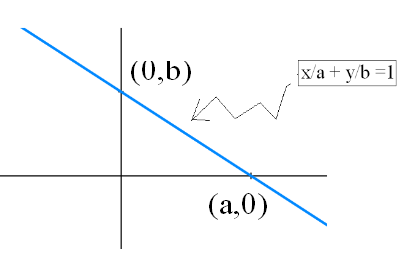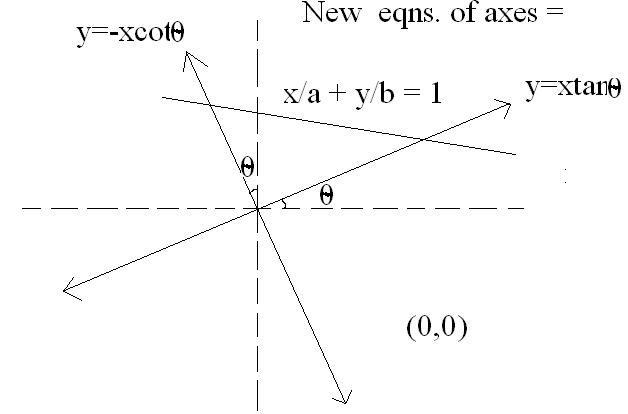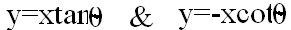# a line has intercepts a and b on the coordinate axis when the axes are rotated through an angle θ,keeping the origin fixed the line makes equal intercepts on the coordinate axes then tanθ=?

10 years agoWhen the axes are rotated through an angle θ,keeping the origin fixed then the graph looks like this:Solving the eqns. x/a + y/b =1 withwe will get,

x = ab/(b + atanθ), y = abtanθ/(atanθ + b)

AND

x = ab/(b - acotθ), y = abcotθ/(acotθ - b) as therir points. of intersections.

Now, it is said that the intercepts made by the line are equal.

Thus, ab/(b + atanθ) = abcotθ/(acotθ - b)

solving this , it will reduce to

tanθ = (a-b)/(a+b)

PLZ APPROVE!

PLZ APPROVE!

PLZ APPROVE!